# 6. Mensuration, Quantitative Aptitude, Civil Service Examination,RPSC Notes | Study RAS RPSC Prelims Preparation - Notes, Study Material & Tests - UPSC

## UPSC: 6. Mensuration, Quantitative Aptitude, Civil Service Examination,RPSC Notes | Study RAS RPSC Prelims Preparation - Notes, Study Material & Tests - UPSC

The document 6. Mensuration, Quantitative Aptitude, Civil Service Examination,RPSC Notes | Study RAS RPSC Prelims Preparation - Notes, Study Material & Tests - UPSC is a part of the UPSC Course RAS RPSC Prelims Preparation - Notes, Study Material & Tests.
All you need of UPSC at this link: UPSC

Mensuration is the branch of mathematics which deals with the study of different geometrical shapes, their areas and Volume. In the broadest sense, it is all about the process of measurement. It is based on the use of algebraic equations and geometric calculations to provide measurement data regarding the width, depth and volume of a given object or group of objects

1. Pythagorean Theorem (Pythagoras' theorem)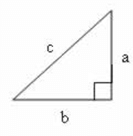In a right angled triangle, the square of the hypotenuse is equal to the sum of the squares of the other two sides

c2 = a2 + b2 where c is the length of the hypotenuse and a and b are the lengths of the other two sides

2. Pi is a mathematical constant which is the ratio of a circle's circumference to its diameter. It is denoted by π

π≈3.14≈227

3. Geometric Shapes and solids and Important Formulas

 Geometric Shapes Description Formulas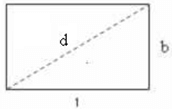Rectanglel = Lengthb = Breadthd= Length of diagonal Area = lbPerimeter = 2(l + b)d = √l2+b2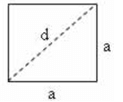Squarea = Length of a sided= Length of diagonal Area= a*a=1/2*d*d                              Perimeter = 4ad = 2√a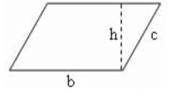Parallelogramb and c are sidesb = baseh = height Area = bhPerimeter = 2(b + c)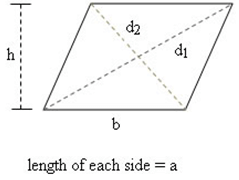Rhombusa = length of each sideb = baseh = heightd1, d2 are the diagonal Area = bh(Formula 1)Area = ½*d1*d2 (Formula 2 )                    Perimeter = 4a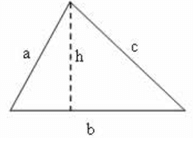Trianglea , b and c are sidesb = baseh = height Area = ½*b*h (Formula 1) Area(Formula 2)                         = √S(S−a)(S−b)(S−c              where S is the semiperimeter       S  =(a+b+c)/2 (Formula 2 for area          -Heron's formula) Perimeter = a + b + cRadius of incircle of a triangle of area A =ASwhere S is the semiperimeter=(a+b+c)/2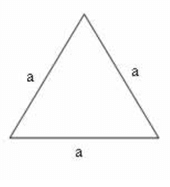Equilateral Trianglea = side Area = (√3/4)*a*a               Perimeter = 3aRadius of incircle of an equilateral                                                                  triangle of side a = a/2*√3Radius of circumcircle of an equilateral triangleof side a = a/√3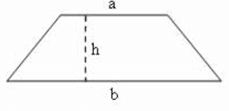Base a is parallel to base b Trapezium (Trapezoid in American English)h = height Area = 12(a+b)h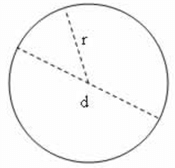Circler = radiusd = diameter d = 2rArea = πr2 =1/4πd2Circumference = 2πr = πd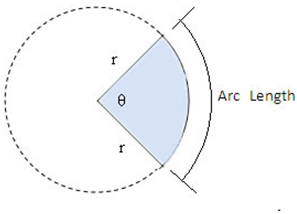Sector of Circler = radiusθ = central angle Area  = (θ/360) *π*r*r  Arc Length, s = (θ/180)* π*rIn the radian system for angular measurement,2π radians = 360°=> 1 radian = 180°π=> 1° = π180 radiansHence,Angle in Degrees= Angle in Radians × 180°πAngle in Radians= Angle in Degrees × π180°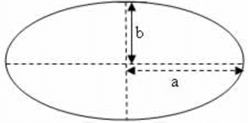EllipseMajor axis length = 2aMinor axis length = 2b Area = πabPerimeter ≈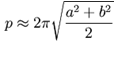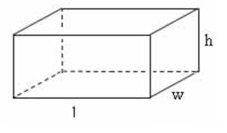Rectangular Solidl = lengthw = widthh = height Total Surface Area= 2lw + 2wh + 2hl= 2(lw + wh + hl)Volume = lwh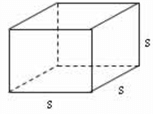Cubes = edge Total Surface Area = 6s2                     Volume = s3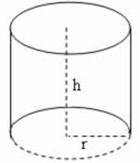Right Circular Cylinderh = heightr = radius of base Lateral Surface Area= (2 π r)hTotal Surface Area= (2 π r)h + 2 (π r2)Volume = (π r2)h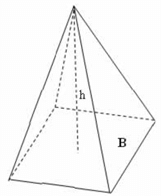Pyramidh = heightB = area of the base Total Surface Area = B + Sum of  the areas of the triangular sidesVolume = 1/3*B*h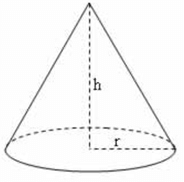Right Circular Coneh = heightr = radius of base Lateral Surface Area=πrswhere s is the slant height =√r*r+h*h Total Surface Area                                =πrs+πr2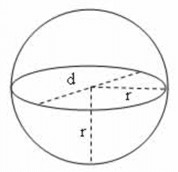Spherer = radiusd = diameter d = 2rSurface Area =4πr*r=πd*dVolume =4/3πr*r*r=16πd*d*d

4. Important properties of Geometric Shapes

a. Properties of Triangle

1. In isosceles triangle, altitude from vertex bisects the base.
2. An isosceles triangle is a triangle with (at least) two equal sides
3. In an equilateral triangle, all three internal angles are each 60°
4. In an equilateral triangle, all three internal angles are congruent to each other
5. An equilateral triangle is a triangle in which all three sides are equal
6. Area of a triangle formed by joining the midpoints of the sides of a given triangle is one-fourth of the area of the given triangle.
7. Centroid divides each median into segments with a 2:1 ratio
8. Centroid is the point where the three medians of a triangle meet.
9. The median of a triangle divides the triangle into two triangles with equal areas
10. The line joining the midpoint of a side of a triangle to the positive vertex is called the median
11. Sum of any two sides of a triangle is greater than the third side.

Sum of the angles of a triangle = 180°

1. The diagonals of a rectangle are equal and bisect each other
2. opposite sides of a rectangle are parallel
3. opposite sides of a rectangle are congruent
4. opposite angles of a rectangle are congruent
5. All four angles of a rectangle are right angles
6. The diagonals of a rectangle are congruent

B. Square

1. All four sides of a square are congruent
2. Opposite sides of a square are parallel
3. The diagonals of a square are equal
4. The diagonals of a square bisect each other at right angles
5. All angles of a square are 90 degrees.
6. A square is a special kind of rectangle where all the sides have equal length

C. Parallelogram

1. The opposite sides of a parallelogram are equal in length.
2. The opposite angles of a parallelogram are congruent (equal measure).
3. The diagonals of a parallelogram bisect each other.
4. Each diagonal of a parallelogram divides it into two triangles of the same area

D. Rhombus

1. All the sides of a rhombus are congruent
2. Opposite sides of a rhombus are parallel.
3. The diagonals of a rhombus bisect each other at right angles
4. Opposite internal angles of a rhombus are congruent (equal in size)
5. Any two consecutive internal angles of a rhombus are supplementary; i.e. the sum of their angles = 180° (equal in size)
6. If each angle of a rhombus is 90°, it is a square

1. The sum of the interior angles of a quadrilateral is 360 degrees
2. If a square and a rhombus lie on the same base, area of the square will be greater than area of the rhombus (In the special case when each angle of the rhombus is 90°, rhombus is also a square and therefore areas will be equal)
3. A parallelogram and a rectangle on the same base and between the same parallels are equal in area.
4. Of all the parallelogram of given sides, the parallelogram which is a rectangle has the greatest area.
5. Each diagonal of a parallelogram divides it into two triangles of the same area
6. A square is a rhombus and a rectangle.

c. Sum of Interior Angles of a polygon

The sum of the interior angles of a polygon = 180(n - 2) degrees where n = number of sides Example 1 : Number of sides of a triangle = 3. Hence, sum of the interior angles of a triangle = 180(3 - 2) = 180 × 1 = 180 ° Example 2 : Number of sides of a quadrilateral = 4. Hence, sum of the interior angles of any quadrilateral = 180(4 - 2) = 180 × 2 = 360.

Solved Examples

1. An error 2% in excess is made while measuring the side of a square. What is the percentage of error in the calculated area of the square?

A. 4.04 %

B. 2.02 %

C. 4 %

D. 2 %

Explanation :

Error = 2% while measuring the side of a square.

Let the correct value of the side of the square = 100
Then the measured value = (100×(100+2))/100=102 (∵ error 2% in excess)

Correct Value of the area of the square = 100 × 100 = 10000
Calculated Value of the area of the square = 102 × 102 = 10404

Error = 10404 - 10000 = 404
Percentage Error = (Error/Actual Value)×100=(404/10000)×100=4.04%

2. A towel, when bleached, lost 20% of its length and 10% of its breadth. What is the percentage of decrease in area?

A. 30 %

B. 28 %

C. 32 %

D. 26 %

Explanation :

Let original length = 100 and original breadth = 100
Then original area = 100 × 100 = 10000

Lost 20% of length
=> New length =( Original length × (100−20))/100

=(100×80)/100=80

=(100×90)/100=90

New area = 80 × 90 = 7200

Decrease in area
= Original Area - New Area
= 10000 - 7200 = 2800

Percentage of decrease in area
=(Decrease in Area/Original Area)×100=(2800/10000)×100=28%

3. If the length of a rectangle is halved and its breadth is tripled, what is the percentage change in its area?

A. 25 % Increase

B. 25 % Decrease

C. 50 % Decrease

D. 50 % Increase

Explanation :

Let original length = 100 and original breadth = 100
Then original area = 100 × 100 = 10000

Length of the rectangle is halved
=> New length = (Original length)/2=100/2=50

=> New breadth= Original breadth × 3 = 100 × 3 = 300

New area = 50 × 300 = 15000

Increase in area = New Area - Original Area = 15000 - 10000= 5000
Percentage of Increase in area =( Increase in Area/OriginalArea)×100=(5000/10000)×100=50%

4. The area of a rectangle plot is 460 square metres. If the length is 15% more than the breadth, what is the breadth of the plot?

A. 14 metres

B. 20 metres

C. 18 metres

D. 12 metres

Explanation :

lb = 460 m2 ------(Equation 1)

Then length, l =( b×(100+15))/100=115b/100------(Equation 2)

From Equation 1 and Equation 2,
115b/100×b=460b2=46000/115=400⇒b=√400=20 m

5. If a square and a rhombus stand on the same base, then what is the ratio of the areas of the square and the rhombus?

A. equal to ½

B. equal to ¾

C. greater than 1

D. equal to 1

Explanation:

If a square and a rhombus lie on the same base, area of the square will be greater than area of the rhombus (In the special case when each angle of the rhombus is 90°, rhombus is also a square and therefore areas will be equal)

Hence greater than 1 is the more suitable choice from the given list

================================================================

Note : Proof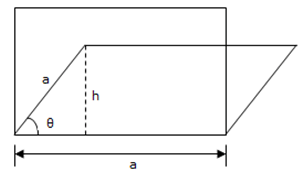Consider a square and rhombus standing on the same base 'a'. All the sides of a square are of equal length. Similarly all the sides of a rhombus are also of equal length. Since both the square and rhombus stands on the same base 'a',

Length of each side of the square = a
Length of each side of the rhombus = a

Area of the sqaure = a2 ...(1)

From the diagram, sin θ = h/a
=> h = a sin θ

Area of the rhombus = ah = a × a sin θ = a2 sin θ ...(2)

From (1) and (2)

Area of the square/Area of the rhombus= a2 /a2sinθ=1/sinθ

Since 0° < θ < 90°, 0 < sin θ < 1. Therefore, area of the square is greater than that of rhombus, provided both stands on same base.

(Note that, when each angle of the rhombus is 90°, rhombus is also a square (can be considered as special case) and in that case, areas will be equal.

6. The breadth of a rectangular field is 60% of its length. If the perimeter of the field is 800 m, find out the area of the field.

A. 37500 m2

B. 30500 m2

C. 32500 m2

D. 40000 m2

Explanation:

Given that breadth of a rectangular field is 60% of its length
b=(60/100)* l =(3/5)* l

perimeter of the field = 800 m
=> 2 (l + b) = 800
⇒2(l+(3/5)* l)=800⇒l+(3/5)* l =400⇒(8/5)* l =400⇒l/5=50⇒l=5×50=250 m

b = (3/5)* l =(3×250)/5=3×50=150 m

Area = lb = 250×150=37500 m2

7. What is the percentage increase in the area of a rectangle, if each of its sides is increased by 20%?

A. 45%

B. 44%

C. 40%

D. 42%

Explanation:

Let original length = 100 and original breadth = 100
Then original area = 100 × 100 = 10000

Increase in 20% of length.
=> New length = (Original length ×(100+20))/100=(100×120)/100=120

New area = 120 × 120 = 14400

Increase in area = New Area - Original Area = 14400 - 10000 = 4400
Percentage increase in area =( Increase in Area /Original Area)×100=(4400/10000)×100=44%

8. What is the least number of squares tiles required to pave the floor of a room 15 m 17 cm long and 9 m 2 cm broad?

A. 814

B. 802

C. 836

D. 900

Explanation:

l = 15 m 17 cm = 1517 cm
b = 9 m 2 cm = 902 cm
Area = 1517 × 902 cm2

Now we need to find out HCF(Highest Common Factor) of 1517 and 902.
Let's find out the HCF using long division method for quicker results

902)  1517  (1

-902

-----------------

615)  902  (1

-       615

-----------------

287)  615 (2

-574

-----------------

41)  287  (7

-287

-----------
0
------------

Hence, HCF of 1517 and 902 = 41

Hence, side length of largest square tile we can take = 41 cm
Area of each square tile = 41 × 41 cm2

Number of tiles required = (1517×902)/(41×41)=37×22=407×2=814

9. A rectangular parking space is marked out by painting three of its sides. If the length of the unpainted side is 9 feet, and the sum of the lengths of the painted sides is 37 feet, find out the area of the parking space in square feet?

A. 126 sq. ft.

B. 64 sq. ft.

C. 100 sq. ft.

D. 102 sq. ft.

Explanation :

Let l = 9 ft.
Then l + 2b = 37
=> 2b = 37 - l = 37 - 9 = 28
=> b = 282 = 14 ft.

Area = lb = 9 × 14 = 126 sq. ft.

10. A large field of 700 hectares is divided into two parts. The difference of the areas of the two parts is one-fifth of the average of the two areas. What is the area of the smaller part in hectares?

A. 400

B. 365

C. 385

D. 315

Explanation :

Let the areas of the parts be x hectares and (700 - x) hectares.

Difference of the areas of the two parts = x - (700 - x) = 2x - 700

one-fifth of the average of the two areas = 15[x+(700−x)]2
=15×7002=3505=70

Given that difference of the areas of the two parts = one-fifth of the average of the two areas
=> 2x - 700 = 70
=> 2x = 770
x=7702=385

Hence, area of smaller part = (700 - x) = (700 – 385) = 315 hectares.

The document 6. Mensuration, Quantitative Aptitude, Civil Service Examination,RPSC Notes | Study RAS RPSC Prelims Preparation - Notes, Study Material & Tests - UPSC is a part of the UPSC Course RAS RPSC Prelims Preparation - Notes, Study Material & Tests.
All you need of UPSC at this link: UPSCUse Code STAYHOME200 and get INR 200 additional OFF

## RAS RPSC Prelims Preparation - Notes, Study Material & Tests

109 docs|21 tests

### How to Prepare for UPSC

Read our guide to prepare for UPSC which is created by Toppers & the best Teachers

Track your progress, build streaks, highlight & save important lessons and more!

,

,

,

,

,

,

,

,

,

,

,

,

,

,

,

,

,

,

,

,

,

,

,

,

,

,

,

,

,

,

,

,

,

;